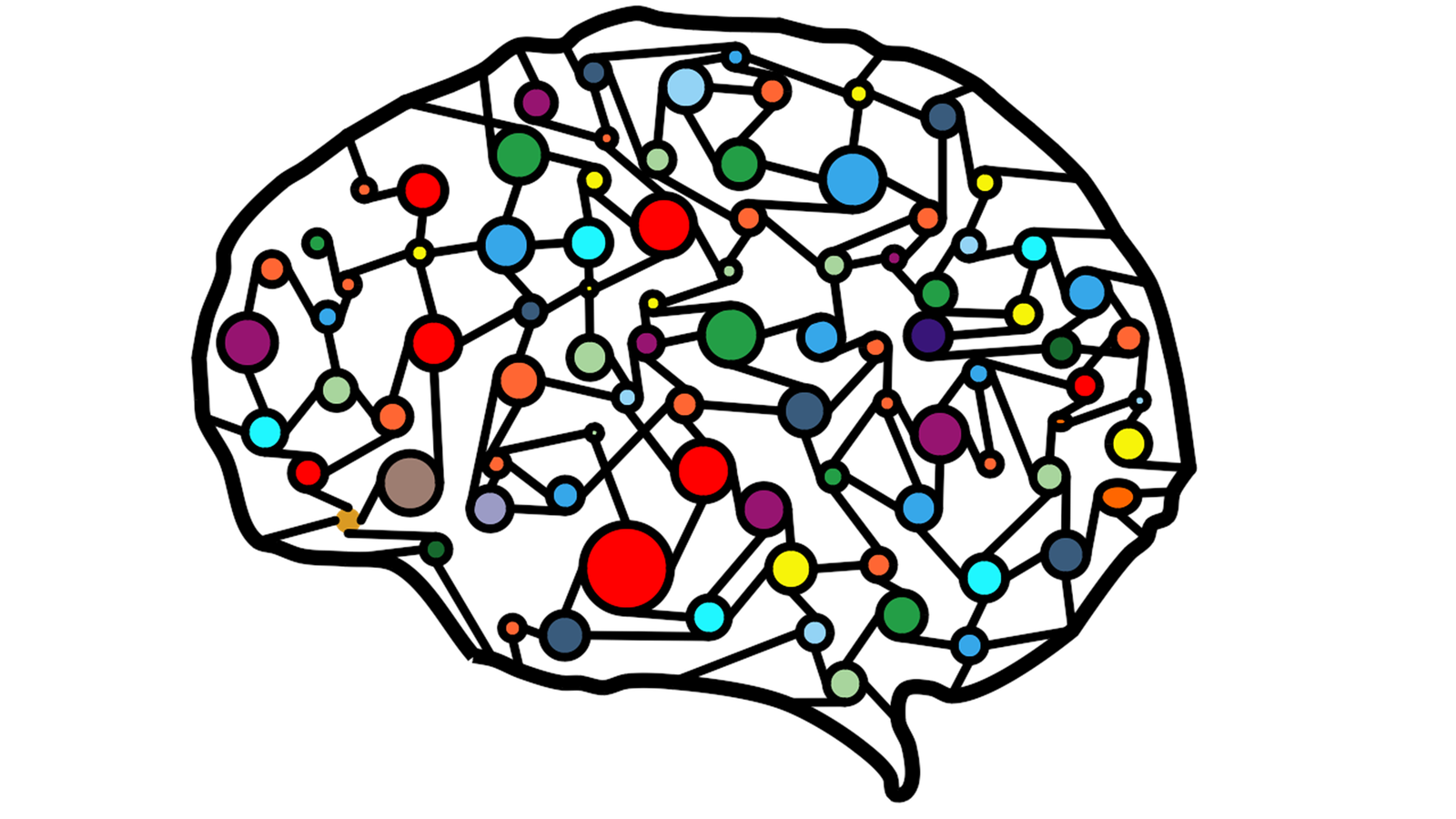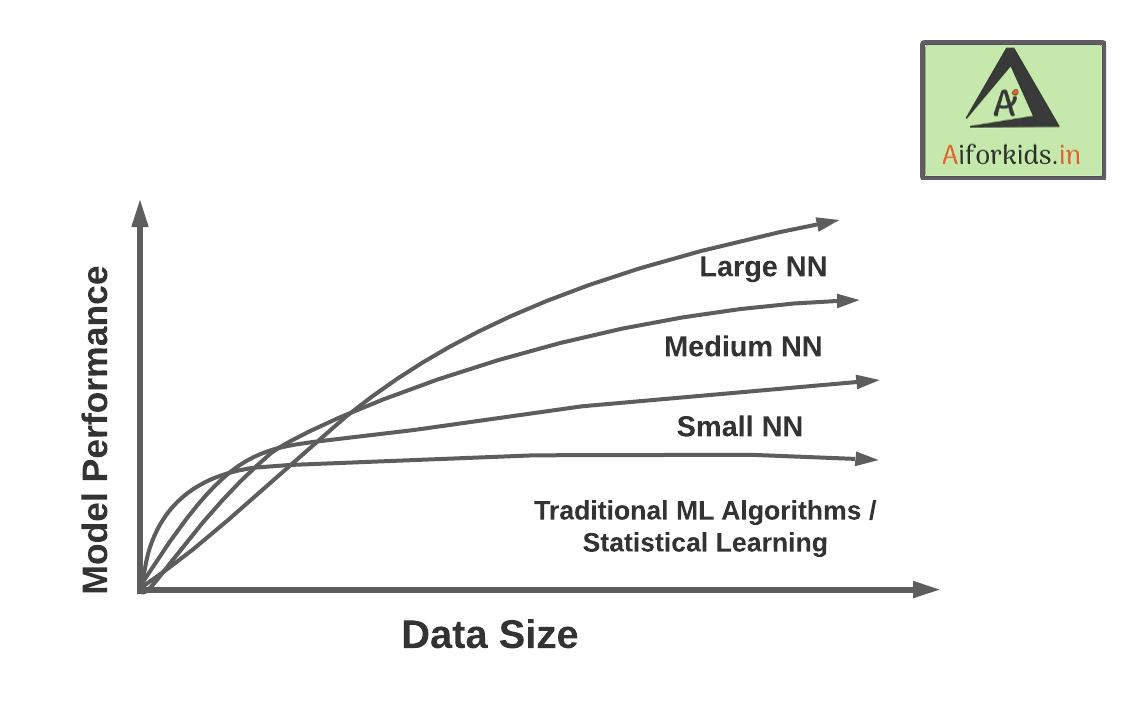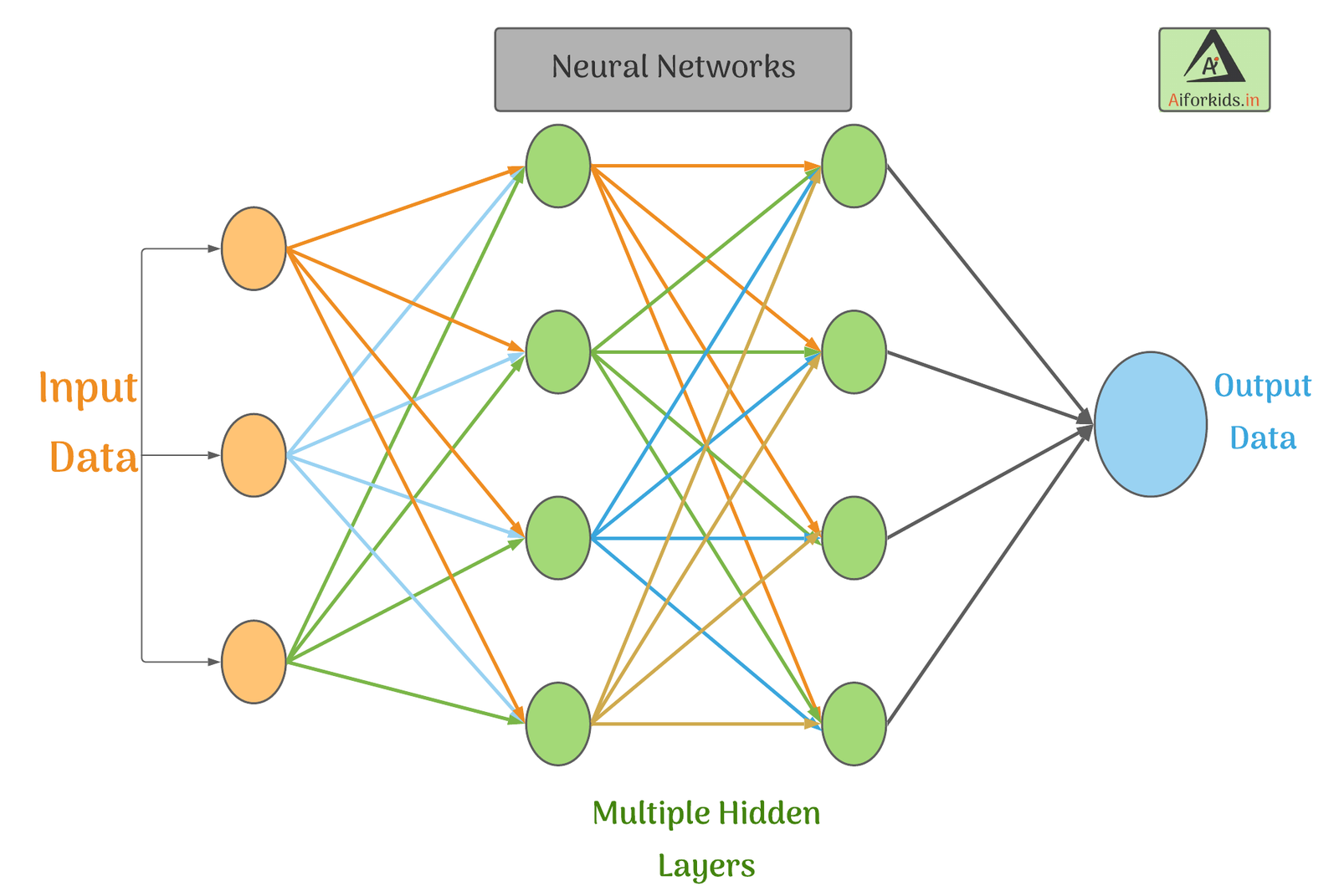## What is Neural Network ?Neural Networks are series of networks of independent Neurons just like in our brain, but in computers, neurons are not the living tissue it is just a algorithm which give output based on given data.

The key advantage of Neural Networks, are that they are able to extract data features automatically without needing the input of the programmer.

A Neural Network is essentially a system of organizing machine learning algorithms to perform certain tasks. It is a fast and efficient way to solve problems for which the dataset is very large, such as in images.

Neural Networks are collection of Neurons containing specific algorithm which are networked together to solve a particular set of problems irrespective of data size

## Why Neural networks ?

To understand clearly, lets dive to its earlier stage!

## If Else Era

In earlier times people tried to solve big data related problems using if else statements, for better understanding let's go through a example.

##### Example:

Suppose we want to build a AI enabled chat app which will talk to people just like any other human. for that we will write if statements (programming concept) checking if he/she says "hello" we will reply "hi" but the problem in this is that we need to write 100 or 1000 lines of if else logic which is very inefficient and a bad practice in programming world. So we take apply concept of our brain to a machine cause we don't have to think "if he says hello i will say hi" it's not a command, the reply changes based on situation.

Neural Networks are base of an AI model. As input data increases the accuracy of Ai increases

#### Solution:

To overcome the disadvantages of if/else statements, people developed neural networks in computer using specific mathematical prediction algorithms.
Due to this we code the AI once and give it a collection of data, which is then processed by these neural networks to give output and the output changes as data changes.

PHP stands for PHP hypertext preprocessor it is a recursive acronym.

## Accuracy-Data Proportionality

The main reason the Neural Networks are implemented to build AI because it learns as it get's more data just like human, if we do something we collect data from surrounding and learn new things and by practicing it (i.e, getting more data on same thing) we get perfect and master it.
To get better idea see the graph given below.As seen in the figure given, the larger Neural Networks tends to perform better with larger amounts of data whereas the traditional machine learning algorithms stop after a certain saturation point.

## How Neural network Works?

This is a representation of how neural networks work. A Neural Network is divided into multiple layers and each layer is further divided into several blocks called nodes.

Each node has its own task to accomplish which is then passed to the next layer. The first layer of a Neural Network is known as the input layer.

The job of an input layer is to acquire data and feed it to the Neural Network. No processing occurs at the input layer. Next to it, are the hidden layers. Hidden layers are the layers in which the whole processing occurs. Their name essentially means that these layers are hidden and are not visible to the user. Each node of these hidden layers has its own machine learning algorithm which it executes on the data received from the input layer. The processed output is then fed to the subsequent hidden layer of the network.

There can be multiple hidden layers in a neural network system and their number depends upon the complexity of the function for which the network has been configured. Also, the number of nodes in each layer can vary accordingly. The last hidden layer passes the final processed data to the output layer which then gives it to the user as the

## Processing Layers in Neural Networks.

#### Input Layer :

The input layer of a neural network is composed of artificial input neurons, and brings the initial data into the system for further processing by subsequent layers of artificial neurons.
The input layer is the very beginning of the workflow for the artificial neural network.

#### Hidden Layer :

A hidden layer in an artificial neural network is a layer in between input layers and output layers, where artificial neurons take in a set of weighted inputs and produce an output through an activation function.
It is a typical part of nearly any neural network in which engineers simulate the types of activity that go on in the human brain.

#### Output Layer :

The output layer is responsible for producing the final result. There must always be one output layer in a neural network.
The output layer takes in the inputs which are passed in from the layers before it, performs the calculations via its neurons and then the output is computed.

The interior layers are sometimes called “hidden layers” because they are not directly observable from the systems inputs and outputs.## Features of Neural Networks:

• Neural Network systems are modelled on the human brain and nervous system.
• They are able to automatically extract features without input from the programmer.
• Every neural network node is essentially a machine learning algorithm.
• It is useful when solving problems for which the data set is very large.

Large ML models require very high computational power which is fullfilled by graphics card in your system.

## Neural Networks vs Human Nervous System

Given are the images of a Human Neuron and its relation with the Neural Network. The axon from a neuron sends an impulse to the synapse of another neuron. The impulse received is then sent to the cell body (nucleus) through dendrites.The cell body performs an activation function on the impulse received and then gives it to the output axon which passes the same to the next neuron in the system. Now as we relate this process with an Artificial Neural Network, we can see that the input layer gets data which is passes on to the nodes in the hidden layer.

The nodes perform specific actions on the data and pass the processed information to the next layer. In the end, the final processed data reaches the output of the system.